# 分享一个自己写的C++代码混淆工具(附源码)

### 分享一个自己写的C++代码混淆工具(附源码)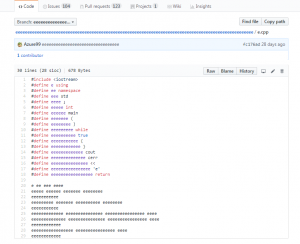C语言中，可以用 #define 定义一个标识符来表示一个常量。其特点是：定义的标识符不占内存，只是一个临时的符号，预编译后这个符号就不存在了

#define 和 #include 一样，也是以“#”开头的。凡是以“#”开头的均为预处理指令，#define也不例外。

#define又称宏定义，标识符为所定义的宏名，简称宏。标识符的命名规则与前面讲的变量的命名规则是一样的。#define 的功能是将标识符定义为其后的常量。一经定义，程序中就可以直接用标识符来表示这个常量。是不是与定义变量类似？但是要区分开！变量名表示的是一个变量，但宏名表示的是一个常量。可以给变量赋值，但绝不能给常量赋值。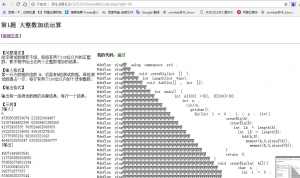1. 先读取cpp代码的源文件，将每一行的内容存放到一个列表中。
2. 将C++里面所有的关键字所有的字符（包括，；. () {} :等等）这些都写到一个列表中去。
3. 在两个for循环中进行遍历，第一重循环遍历源码的每一行，第二重循环遍历查找是否有字符列表中的字符，如果有就进行替换。再写入到新文件中去。
4. 在ascii码表中找一个范围，随机生成一个首字母来作为替换的内容。
5. 再加入一些随机的注释，让混淆后的代码看起来更乱。

```import random
import string

keyword_list = ['cout','+=','-=','int ','goto','asm', 'do', 'if','[',']',
'return', 'typedef', 'auto', 'double', 'inline','{','}',
'short', 'typeid', 'bool', 'int ','(',')',
'signed', 'typename', 'break', 'else','&gt;=','&lt;=',
'sizeof', 'union', 'case', 'enum', 'mutable',';',
'static', 'unsigned', 'catch', 'explicit', 'try',
'namespace', 'using', 'char','main','const',
'export', 'new', 'struct', 'class', 'switch',
'false', 'private', 'long','::', 'void','endl',
'float', 'protected', 'this', 'continue','++','--',
'for', 'public', 'throw', 'while', 'default', 'friend',
'true','&lt;&lt;','cin','printf','==','&gt;&gt;','!=',]

def random_char():
r = chr(random.randint(97,122))
char,char_r,list_chr = [],[],[]
for i in range(len(keyword_list)):
char.append(r+str(i))
char_r.append(keyword_list[i])
random.shuffle(char)
random.shuffle(char_r)
for i in range(len(char)):
list_chr.append([char[i],char_r[i]])
return list_chr

def generate_define(list_chr):
define = []
for i in range(len(list_chr)):
define.append('#define '+ list_chr[i] +' '+list_chr[i])
return define

def replace(list_char,str,confusion):
if confusion == ' /**/ ':
confusion = ' /*' + ''.join(random.sample(string.hexdigits,6)) +'*/ '
for i in list_char:
if i in str:
str = str.replace(i,confusion+i+confusion)
return str

def open_file(list_char):
cpp = input('请输入一个C++代码的文件名:')
confusion = input('请输入混淆注释（可选,不填则随机混淆）:')
confusion = ' /*' + confusion + '*/ '
filenmae = cpp.split('.')+'混淆.cpp'
with open(cpp,'r') as f:
with open(filenmae, 'w') as m:
define = generate_define(list_char)
for i in define:
m.write(i+'\n')
for i in f.readlines():
if '#' in i:
m.write(i)
continue
i=i.strip()
i_replace = replace(list_char,i,confusion)
m.write(i_replace+'\n')
print('混淆代码完成了哦^_^！\n混淆后的文件名为： '+filenmae+'\n')

if __name__ =='__main__':
print('-' * 60)
print('          C++ 代码混淆工具beta版        Author By: Zgao\n')
print('                  tips: cpp代码和该软件需在同一目录下\n')
print('-'*60)
while True:
open_file(random_char())```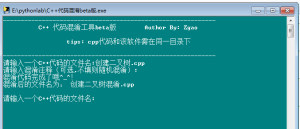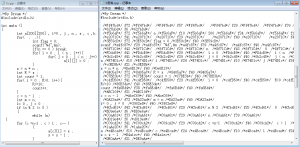微信赞赏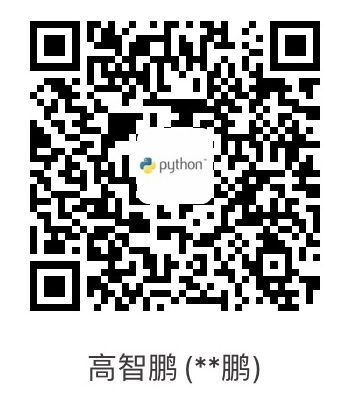支付宝赞赏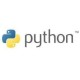### 5条评论###### alert(1) 发布于5:37 下午 - 11月 6, 2020###### 匿名 发布于11:08 下午 - 5月 4, 2019

grep -v “#include” e.c | gcc -E – | grep -v “#” | indent